Most Affordable JEE | NEET | 8,9,10 Preparation by Kota's Top IITian Doctor Faculties

# NCERT Solutions for Class 9 Maths chapter 4 Exercise 4.3 - Linear Equations in Two Variables

Class 9`

Hey, are you a class 9 Student and Looking for Ways to Download NCERT Solutions for Class 9 Maths chapter 4 Exercise 4.3? If Yes then you are at the right place.

Here we have listed Class 9 maths chapter 4 exercise 4.3 solutions in PDF that is prepared by Kota’s top IITian’s Faculties by keeping Simplicity in mind.

If you want to score high in your class 9 Maths Exam then it is very important for you to have a good knowledge of all the important topics, so to learn and practice those topics you can use eSaral NCERT Solutions.

So, without wasting more time Let’s start.

### Download The PDF of NCERT Solutions for Class 9 Maths chapter 4 Exercise 4.3 "Linear Equations in Two Variables"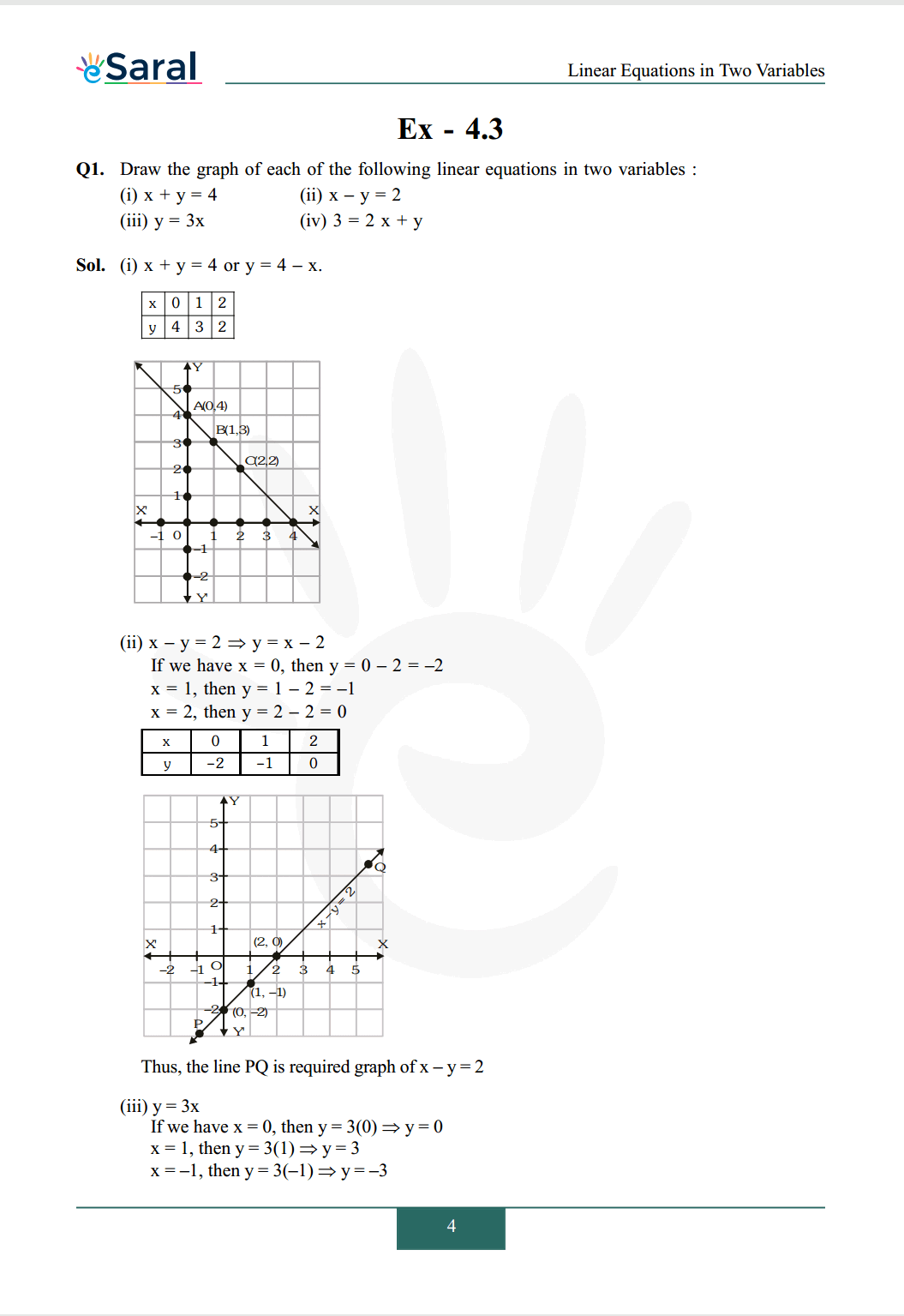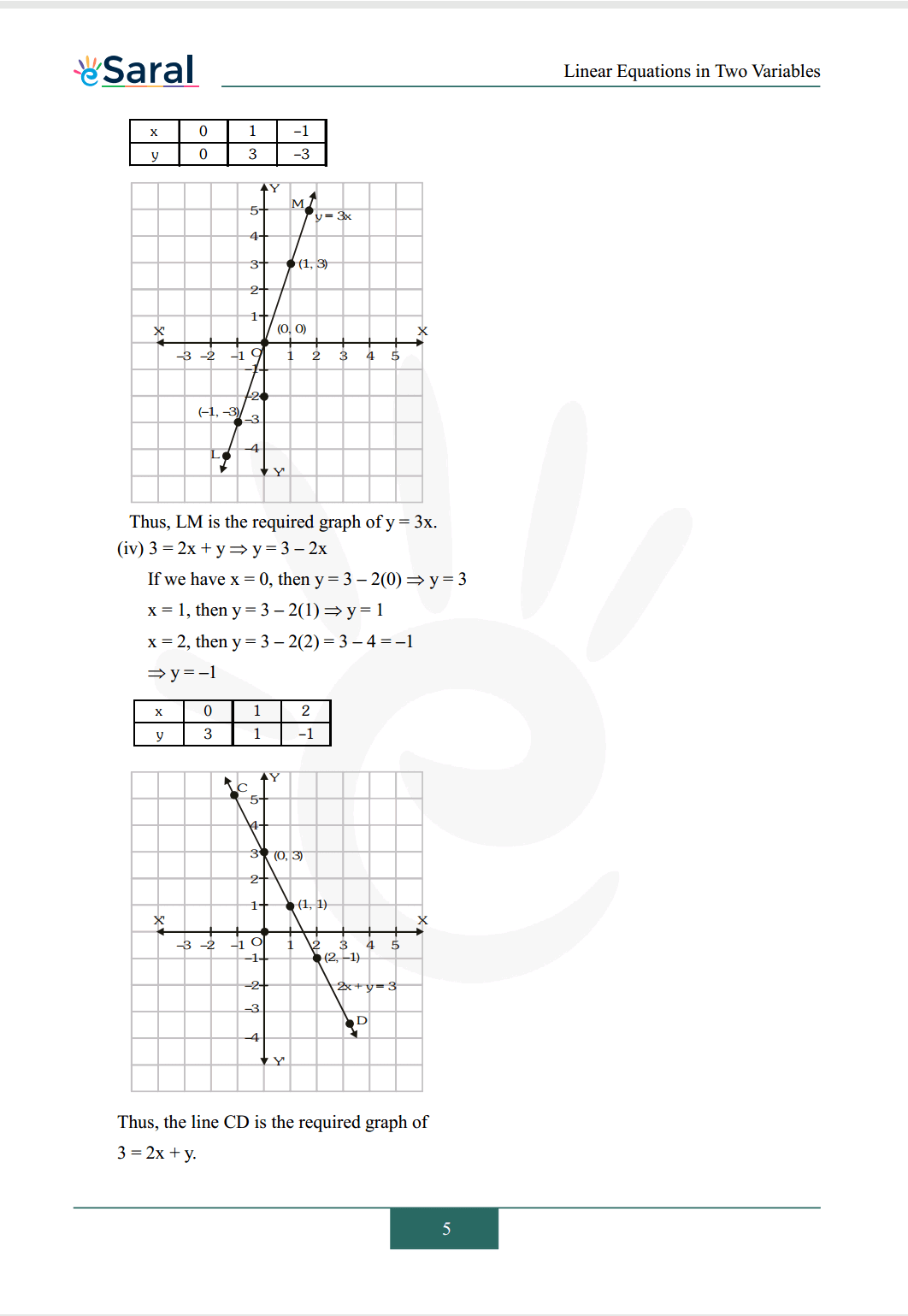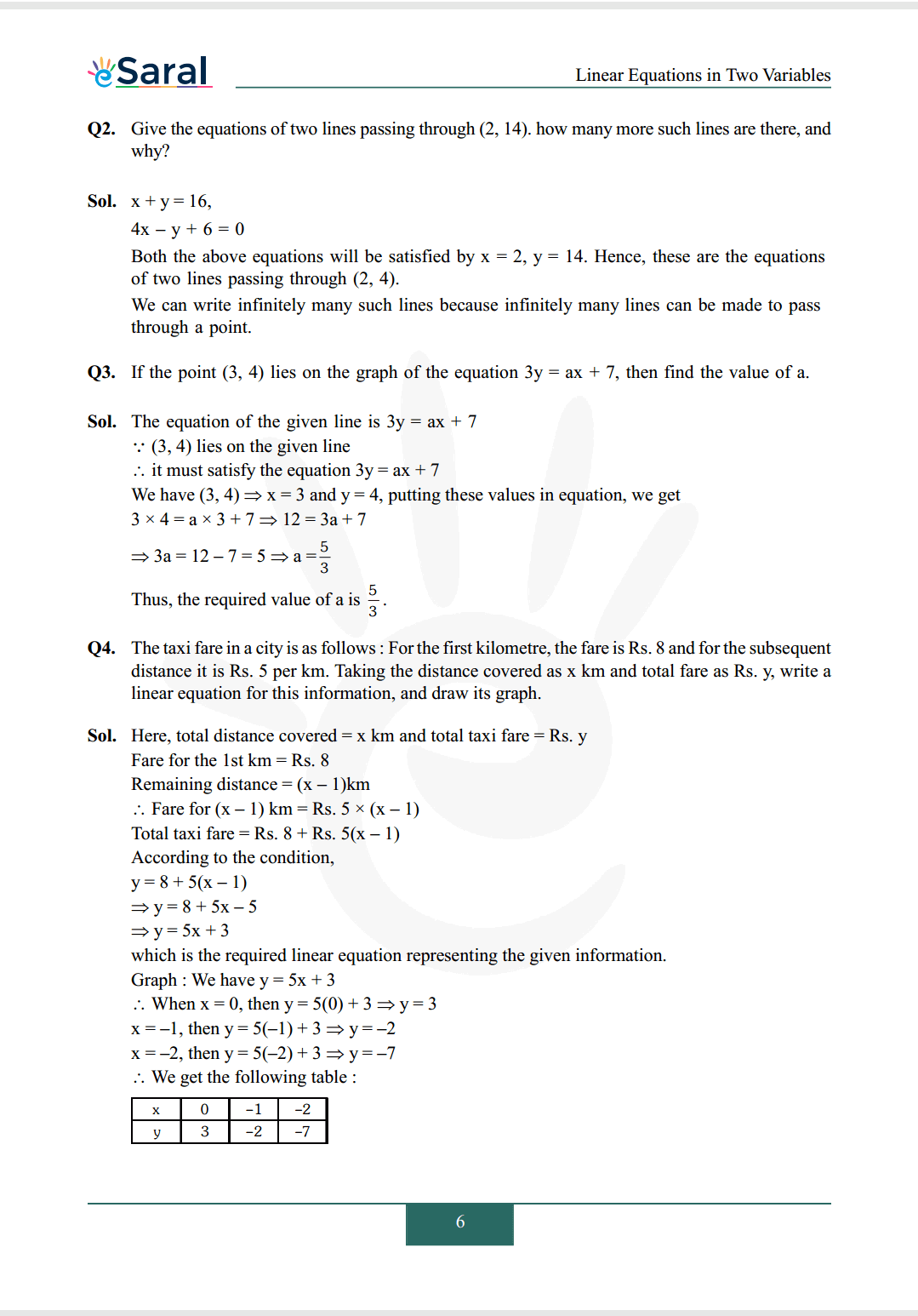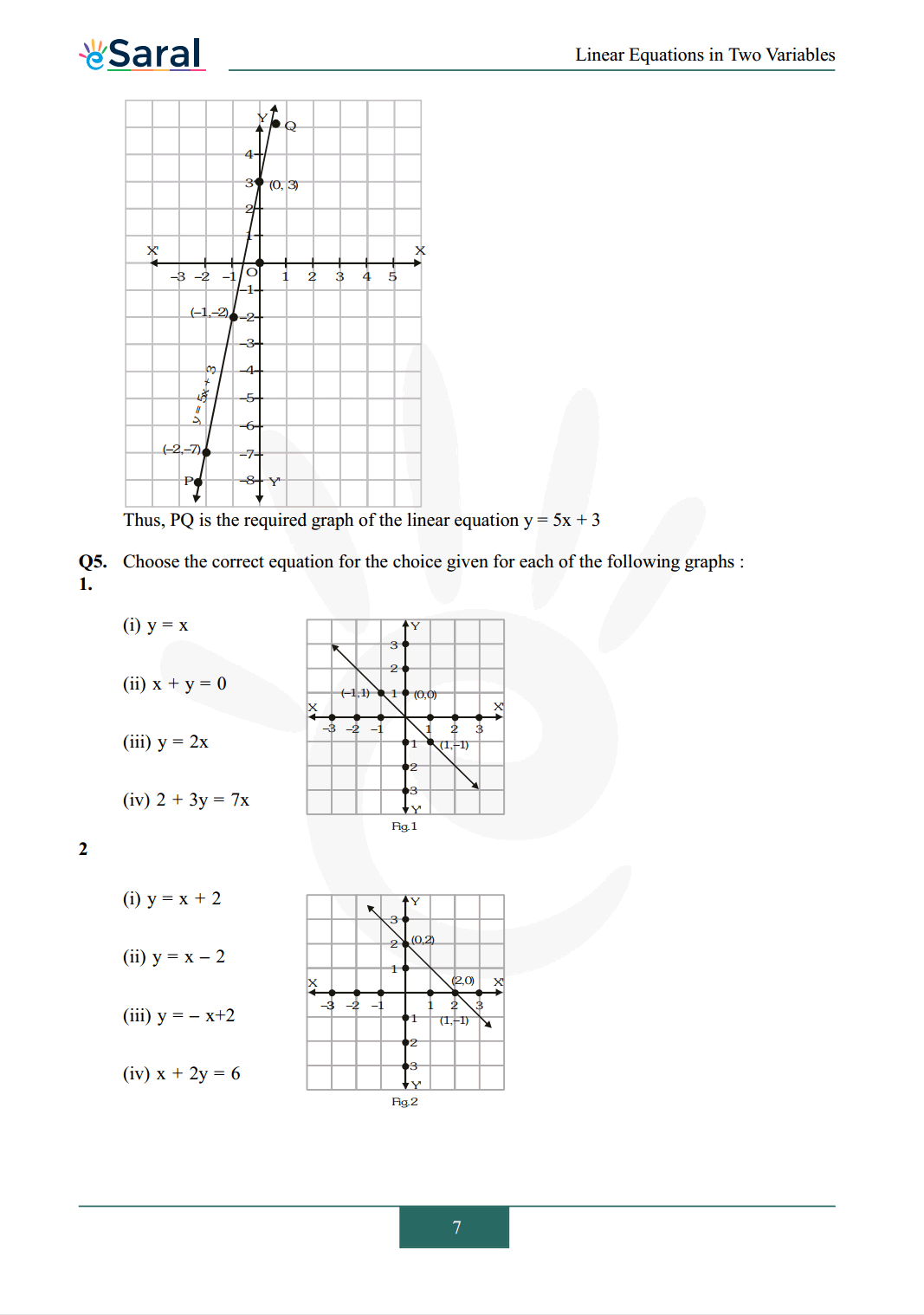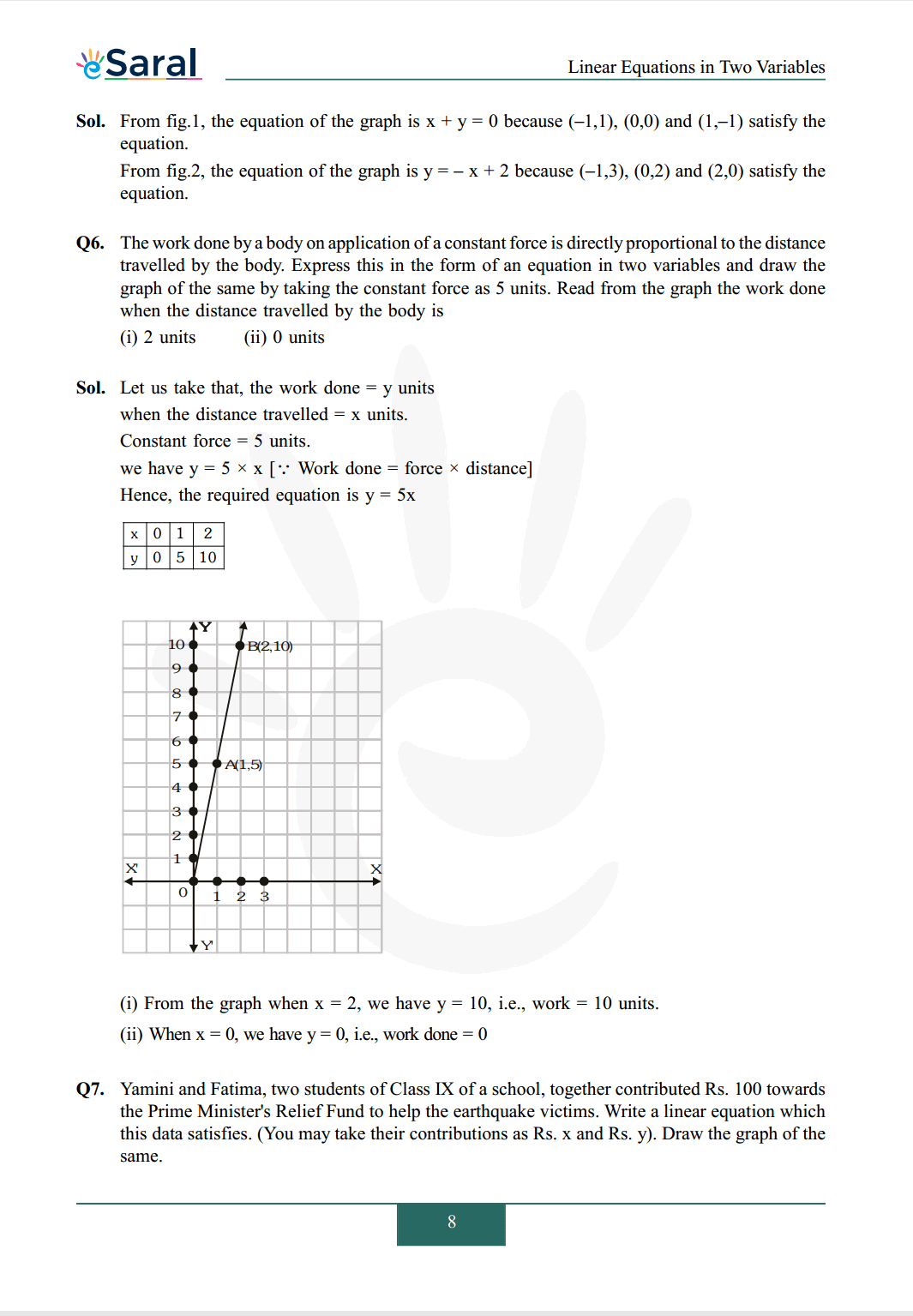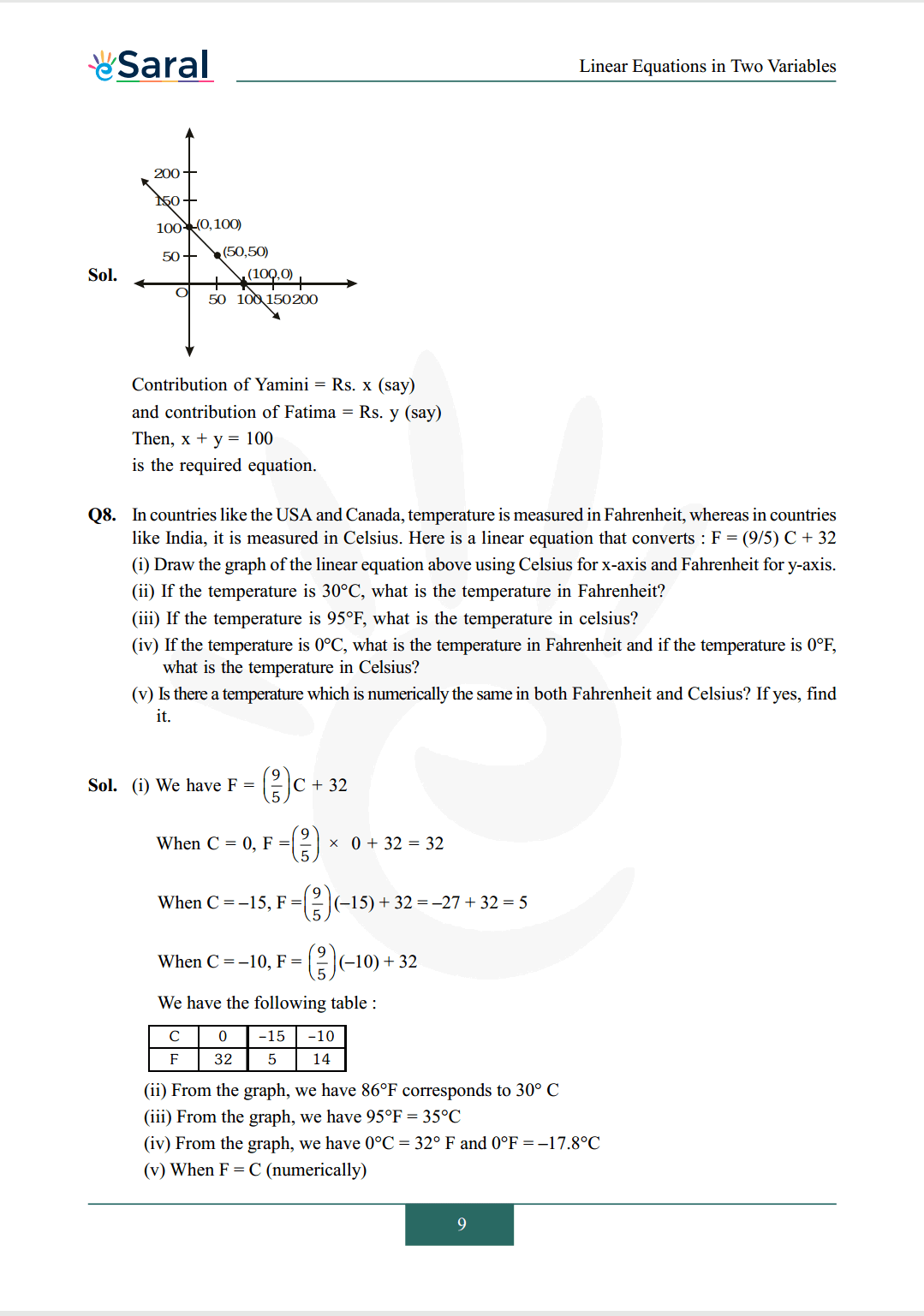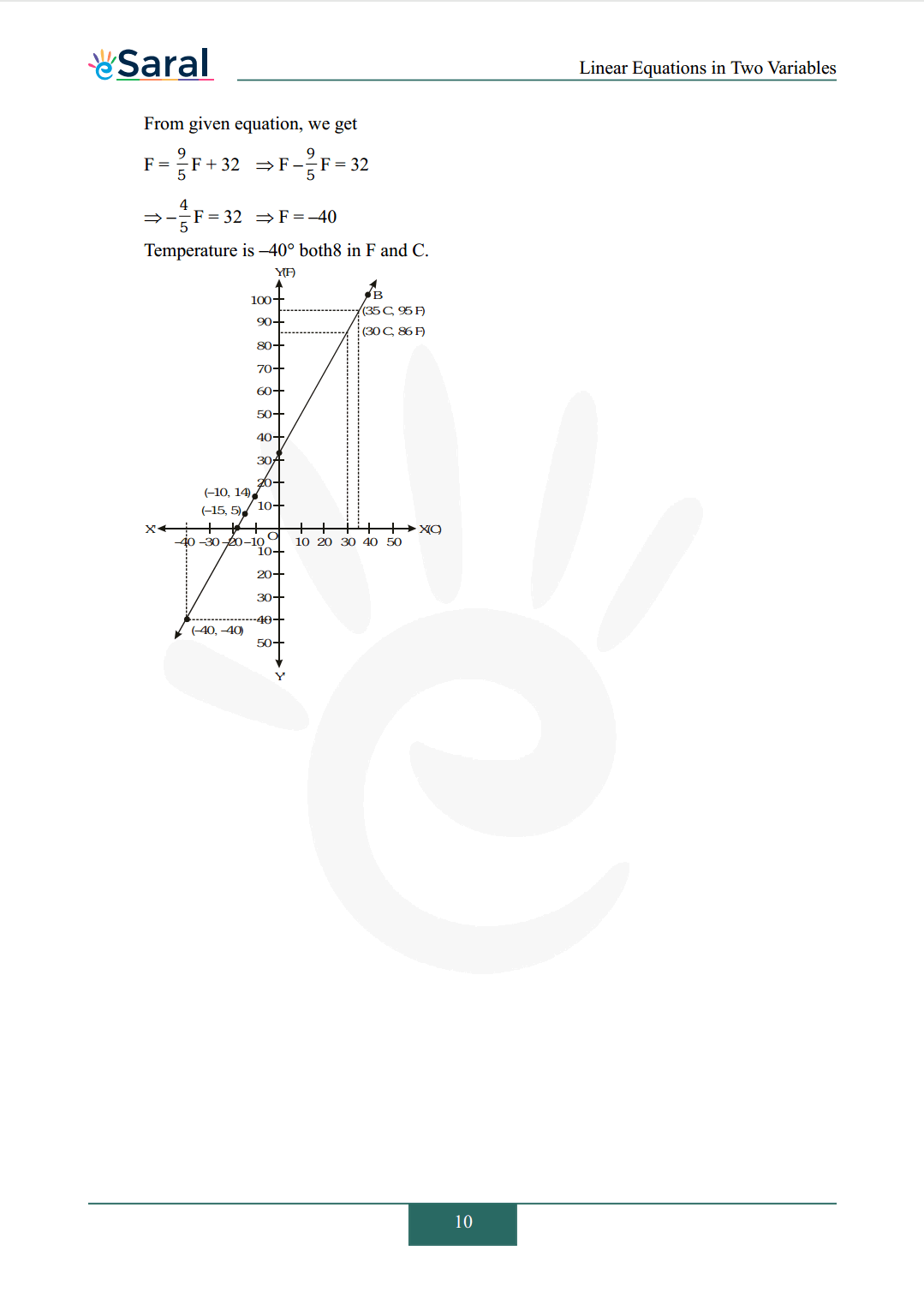All Questions of Chapter 4 Exercise 4.3

Once you complete the chapter 4 then you can revise Ex. 4.3 by solving following questions

Q1. Draw the graph of each of the following linear equations in two variables :
(i) $x+y=4$
(ii) $x-y=2$
(iii) $\mathrm{y}=3 \mathrm{x}$
(iv) $3=2 \mathrm{x}+\mathrm{y}$

Q2. Give the equations of two lines passing through $(2,14)$. how many more such lines are there, and why?

Q3. If the point $(3,4)$ lies on the graph of the equation $3 \mathrm{y}=\mathrm{ax}+7$, then find the value of a.

Q4. The taxi fare in a city is as follows: For the first kilometre, the fare is Rs. 8 and for the subsequent distance it is Rs. 5 per km. Taking the distance covered as x km and total fare as Rs. y, write a linear equation for this information, and draw its graph.

Q5. Choose the correct equation for the choice given for each of the following graphs :

1.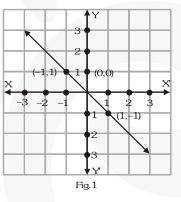(i) $\mathrm{y}=\mathrm{x}$
(ii) $x+y=0$
(iii) $\mathrm{y}=2 \mathrm{x}$
(iv) $2+3 y=7 x$

2.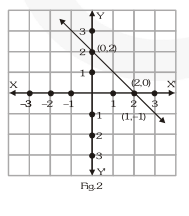(i) $\mathrm{y}=\mathrm{x}+2$
(ii) $y=x-2$
(iii) $\mathrm{y}=-\mathrm{x}+2$
(iv) $x+2 y=6$

Q6. The work done by a body on application of a constant force is directly proportional to the distance travelled by the body. Express this in the form of an equation in two variables and draw the graph of the same by taking the constant force as 5 units. Read from the graph the work done when the distance travelled by the body is
(i) 2 units
(ii) 0 units

Q7. Yamini and Fatima, two students of Class IX of a school, together contributed Rs. 100 towards the Prime Minister's Relief Fund to help the earthquake victims. Write a linear equation which this data satisfies. (You may take their contributions as Rs. $\mathrm{x}$ and Rs. y). Draw the graph of the same.

Q8. In countries like the USA and Canada, temperature is measured in Fahrenheit, whereas in countries like India, it is measured in Celsius. Here is a linear equation that converts : $\mathrm{F}=(9 / 5) \mathrm{C}+32$
(i) Draw the graph of the linear equation above using Celsius for $\mathrm{x}$ -axis and Fahrenheit for $\mathrm{y}$ -axis.
(ii) If the temperature is $30^{\circ} \mathrm{C}$, what is the temperature in Fahrenheit?
(iii) If the temperature is $95^{\circ} \mathrm{F}$, what is the temperature in celsius?
(iv) If the temperature is $0^{\circ} \mathrm{C}$, what is the temperature in Fahrenheit and if the temperature is $0^{\circ} \mathrm{F}$, what is the temperature in Celsius?
(v) Is there a temperature which is numerically the same in both Fahrenheit and Celsius? If yes, find it.

Class 9 NCERT Maths Book Chapterwise

Class 9 NCERT Maths Exemplar Chapterwise